# 1 Introduction

## 1.1 Installation

Currently, barcodetrackR is available at Github and can be downloaded using the devtools package.

``devtools::install_github("dunbarlabNIH/barcodetrackR")``

## 1.2 Contributors

The R package and functions were created by Diego A. Espinoza, Ryland D. Mortlock, Samson J. Koelle, and others at Cynthia Dunbar’s laboratory at the National Heart, Lung, and Blood Institutes of Health. Issues should be addressed to https://github.com/dunbarlabNIH/barcodetrackR/issues.

The barcodetrackR package operates on SummarizedExperiment objects from the Bioconductor repository. It stores associated colData for each sample in this object as well as any metadata. We load the `barcodetrackR` and `SummarizedExperiment` packages here for our analyses, as well as the `magrittr` package in order to improve legibility of code through using the pipe `%>%` operator.

``````require("magrittr")
require("barcodetrackR")
require("SummarizedExperiment")``````

## 2.2 Creating objects with `create_SE`

For this vignette, we will load publically available data from the following papers (these sample datasets are included in the R package):

``````system.file("extdata", "/WuC_etal_appdata/sample_data_ZJ31.txt", package = "barcodetrackR") %>%

system.file("extdata", "/WuC_etal_appdata/sample_metadata_ZJ31.txt", package = "barcodetrackR") %>%

wu_SE <- create_SE(your_data = wu_dataframe,
threshold = 0)

system.file("extdata", "/BelderbosME_etal/count_matrix_mouse_C21.txt", package = "barcodetrackR") %>%

system.file("extdata", "/BelderbosME_etal/metadata_mouse_C21.txt", package = "barcodetrackR") %>%

belderbos_SE <- create_SE(your_data = belderbos_dataframe,
threshold = 0)

system.file("extdata", "/SixE_etal/WAS5_reads.txt", package = "barcodetrackR") %>%

system.file("extdata", "/SixE_etal/WAS5_metadata.txt", package = "barcodetrackR") %>%

six_SE <- create_SE(your_data = six_dataframe,
threshold = 0)``````
``````## No threshold supplied. All barcodes will be retained. Be aware that lower abundance barcodes are likely to be less reliable due to sampling bias. To estimate an appropriate threshold, please see the barcodetrackR function `estimate_barcode_threshold`.
## No threshold supplied. All barcodes will be retained. Be aware that lower abundance barcodes are likely to be less reliable due to sampling bias. To estimate an appropriate threshold, please see the barcodetrackR function `estimate_barcode_threshold`.
## No threshold supplied. All barcodes will be retained. Be aware that lower abundance barcodes are likely to be less reliable due to sampling bias. To estimate an appropriate threshold, please see the barcodetrackR function `estimate_barcode_threshold`.``````

Our input dataframes to create the `SummarizedExperiment` (SE) objects are each an n x m `data.frame` where there are n rows of observations (typically cellular barcodes, insertion sites, or the like) and the m columns are the samples. The input metadata must have row order identical to the order of the colums in its corresponding dataframe. The metadata must also have a column titled `SAMPLENAME` that denotes the column of `your_data` it refers to.

## 2.3 Assays created by `create_SE`

`create_SE` takes the input `dataframe` and metadata and creates a SummarizedExperiment object with the following assays:

• `counts`: the raw values from the input dataframe
• `proportions`: the per-column proportions of each entry in each column
• `ranks`: the rank of each entry in each column
• `normalized`: the normalized read values (CPM)
• `logs`: the log of the normalized values
``assays(six_SE)``
``````## List of length 5
## names(5): counts proportions ranks normalized logs``````

## 2.4 Thresholding barcoding data

We also include a function to help users estimate the minimum abundance of reliable barcodes. For a specified capture efficiency C, the minimum clone size N that we can expect to detect with confidence level P is calculated from:

\(P = 1 - (1-C)^N\)

The proportional abundance of a clonal tag of size N is:

\(N/(T * F)\)

where T is the total population size of cells or genomes and F is the frequency or proportion of the total population which is labeled or genetically modified with the clonal tag.

The population size and proportion labeled must be determined experimentally. The capture efficiency should be estimated for a given clonal tracking technique by simulating the barcode retrieval process in silico and finding the capture efficiency which leads to a total # of detected barcodes matching the experimentally determined number. Adair et al (PMID: 32355868) performed this analysis for viral integration site analysis and DNA barcode sequencing and determined good estimates for the capture efficiencies of these two technologies to be 0.05 and 0.4 respectively.

``````my_thresh <- estimate_barcode_threshold(capture_efficiency = 0.4,
population_size = 500000,
proportion_labeled = 0.3,
confidence_level = 0.95,
verbose = TRUE)``````
``## Relative threshold. Barcodes above 0.003909661 % of a given sample are estimated to be reliable.``

This threshold can be applied to an existing SE to remove low abundance barcodes which do not reach the thresold in any sample.

``````wu_thresh <- threshold_SE(your_SE = wu_SE,
threshold_value = 0.005,
threshold_type = "relative",
verbose = TRUE)``````
``## Removed 35353 barcodes from the supplied dataframe based on relative threshold of 0.005``

Users can also specify an absolute threshold count rather than a relative threshold by changing the `threshold_type` to “absolute”.

# 3 Shiny app

The barcodetrackR package includes a Shiny app for users without programming experience to analyze clonal tracking data and create high-quality visualizations. To launch the app, use the following line of code.

``barcodetrackR::launchApp()``

# 4 Correlations

## 4.1`scatter_plot`

A straightforward way to view the relationship between samples in a pairwise manner is to view basic scatter plots of two samples using the provided assays. We provide a `scatter_plot` function as part of the package.

Here, we view the correlation of barcode “proportions” between different cell types at the 20 month timepoint of the Wu et al study. We compare granulocytes (Gr) to B and T cells.

``````Gr_B_20 <- c("ZJ31_20m_Gr", "ZJ31_20m_B")
Gr_T_20 <- c("ZJ31_20m_Gr", "ZJ31_20m_T")
wu_scatterplot_1 <- scatter_plot(wu_SE[,Gr_B_20], your_title = "Gr vs B", assay = "proportions")
wu_scatterplot_2 <- scatter_plot(wu_SE[,Gr_T_20], your_title = "Gr vs T", assay = "proportions")
cowplot::plot_grid(wu_scatterplot_1, wu_scatterplot_2, ncol = 2)``````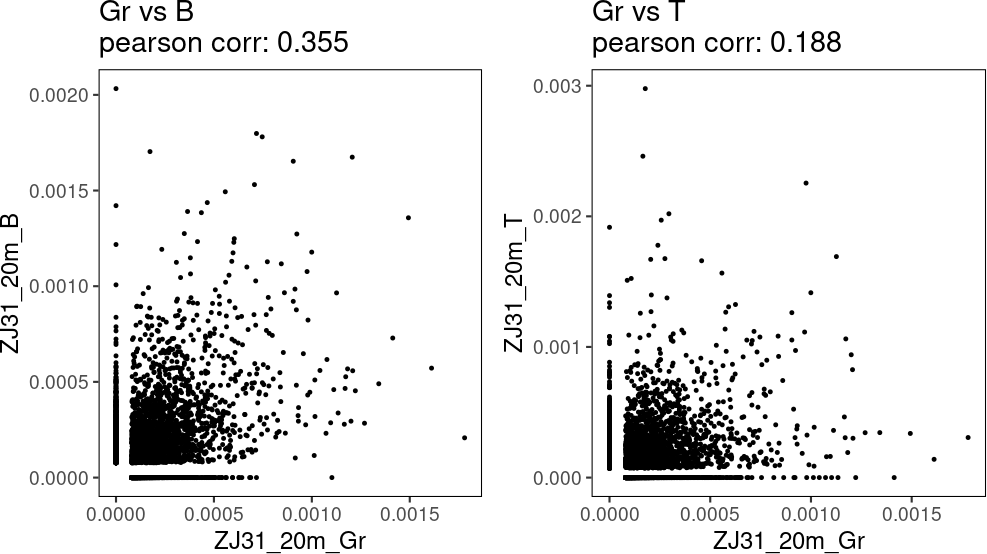## 4.2`cor_plot`

A more comprehensive way to view the relationship between samples in a pairwise manner is to use a correlation plot. Here, we visualize the Pearson correlation of the barcode “proportions” between the T, B, Gr, NK CD56+/CD16-, and NK CD56-/CD16+ fractions within the Wu dataset for the 6, 9.5, 12, and 20 month post-transplant timepoints.

``````wu_cor_plot_sample_selection <- colData(wu_SE)\$SAMPLENAME[1:20]
cor_plot(wu_SE[,wu_cor_plot_sample_selection],
method_corr = "pearson",
plot_type = "color",
assay = "proportions")``````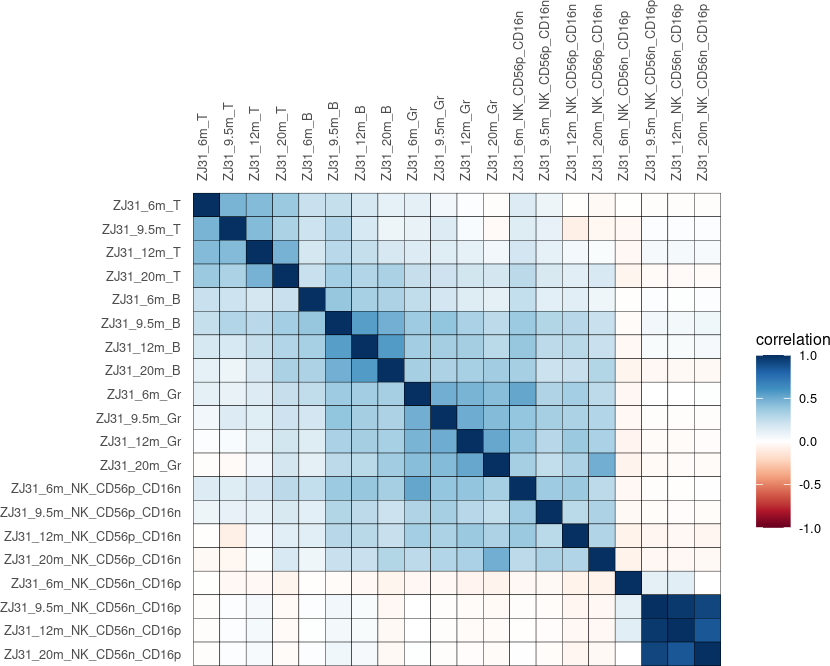If desired, we can also calculate and visualize the Pearson correlations for the “logs” assay for the same samples above.

``````wu_cor_plot_sample_selection <- colData(wu_SE)\$SAMPLENAME[1:20]
cor_plot(wu_SE[,wu_cor_plot_sample_selection],
method_corr = "pearson",
plot_type = "color",
assay = "logs")``````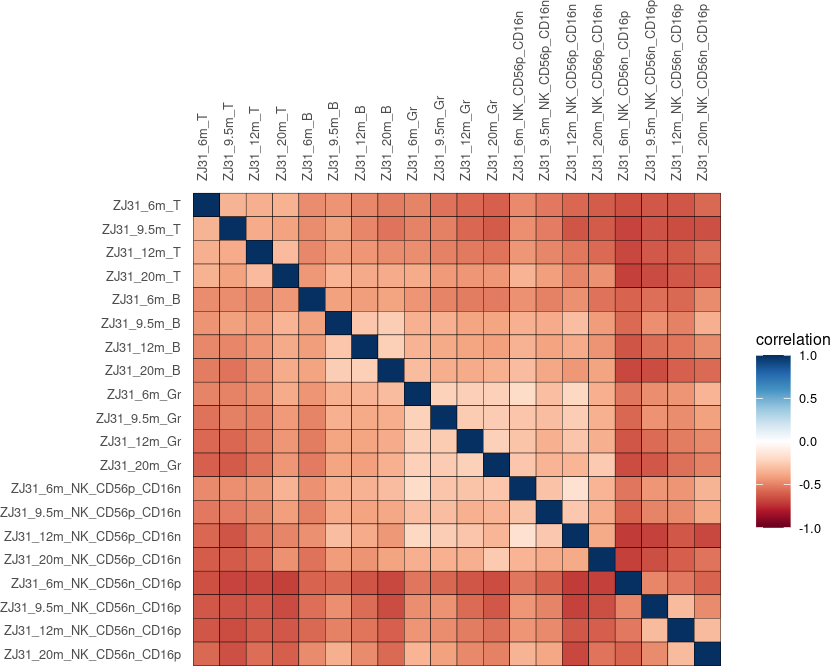We can return a table of the Pearson correlations as well as the p-values and confidence intervals for each of the comparisons above. This argument `"return_table"` is included in all barcodetrackR functions which conduct mathematical or statistical analysis. By setting the option to “TRUE”, users can return the calculated data as a dataframe rather than display the visualization. Here, we return the p-values and confidence intervals for the correlations calculated using the “proportions” assay.

``````cor_plot(wu_SE[,wu_cor_plot_sample_selection],
method_corr = "pearson",
assay = "proportions",
``````##       sample_i    sample_j correlation_value       p_value     ci_lo     ci_hi
## cor  ZJ31_6m_T   ZJ31_6m_T         1.0000000  0.000000e+00 1.0000000 1.0000000
## cor1 ZJ31_6m_T ZJ31_9.5m_T         0.4672994  0.000000e+00 0.4527245 0.4816246
## cor2 ZJ31_6m_T  ZJ31_12m_T         0.4406121  0.000000e+00 0.4261147 0.4548832
## cor3 ZJ31_6m_T  ZJ31_20m_T         0.3730234  0.000000e+00 0.3564373 0.3893744
## cor4 ZJ31_6m_T   ZJ31_6m_B         0.2263901 7.739959e-201 0.2122372 0.2404479
## cor5 ZJ31_6m_T ZJ31_9.5m_B         0.2346975 2.001192e-191 0.2197056 0.2495785``````

Above, we used two of the three available correlation visualizations (`"color"` and `"circle"`) using the standard color palette provided. A `no_negative` parameter is offered as well to set all negative correlations in the data to equal 0. This may be done to eliminate negative correlations from the data, from which deriving biological meaning may be difficult.

When there are a smaller number of samples to analyze, the `"number"` option can be used to view the actual correlation within the grid. Here is an example visualizing the Pearson correlations of peripheral blood samples at subsequent timepoints from the Belderbos et al sample data set. Note: within these samples, U stands for unsorted.

``````belderbos_wk_samples_PB <- c("wk9_U", "wk14_U", "wk20_U", "wk22_U")
cor_plot(your_SE = belderbos_SE[,belderbos_wk_samples_PB],
method_corr = "pearson",
plot_type = "number",
assay = "proportions",
number_size = 3)``````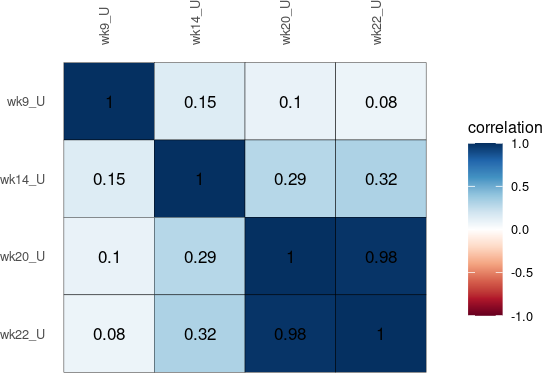# 5 Distances and similarities

## 5.1`dist_plot`

While correlation coefficients provide some insight into pairwise comparisons between samples, there exist a number of measures and metrics possible for pairwise sample comparisons (namely, distances and similarities). The `proxy` package provides a number of distance and similarity measure we can incorporate for our purposes.

``summary(proxy::pr_DB)``
``````## * Similarity measures:
## Braun-Blanquet, Chi-squared, Cramer, Dice, Fager, Faith, Gower, Hamman,
## Jaccard, Kulczynski1, Kulczynski2, Michael, Mountford, Mozley, Ochiai,
## Pearson, Phi, Phi-squared, Russel, Simpson, Stiles, Tanimoto,
## Tschuprow, Yule, Yule2, correlation, cosine, eDice, eJaccard, simple
## matching
##
## * Distance measures:
## Bhjattacharyya, Bray, Canberra, Chord, Euclidean, Geodesic, Hellinger,
## Kullback, Levenshtein, Mahalanobis, Manhattan, Minkowski, Podani,
## Soergel, Wave, Whittaker, divergence, fJaccard, supremum``````

We will use the Wu dataset as in the correlation plots above, this time using the `logs` assay to determine pairwise sample distances or similarities. The use of a similarity or distance will be automatically detected in the argument `dist_method` and plotted appropriately. The samples may be clustered or not, using the `cluster_tree` argument. We first show the Manhattan distances calculated between samples for the Wu dataset.

``````dist_plot(wu_SE[,wu_cor_plot_sample_selection],
dist_method =  "manhattan",
plot_type = "color",
assay = "logs")``````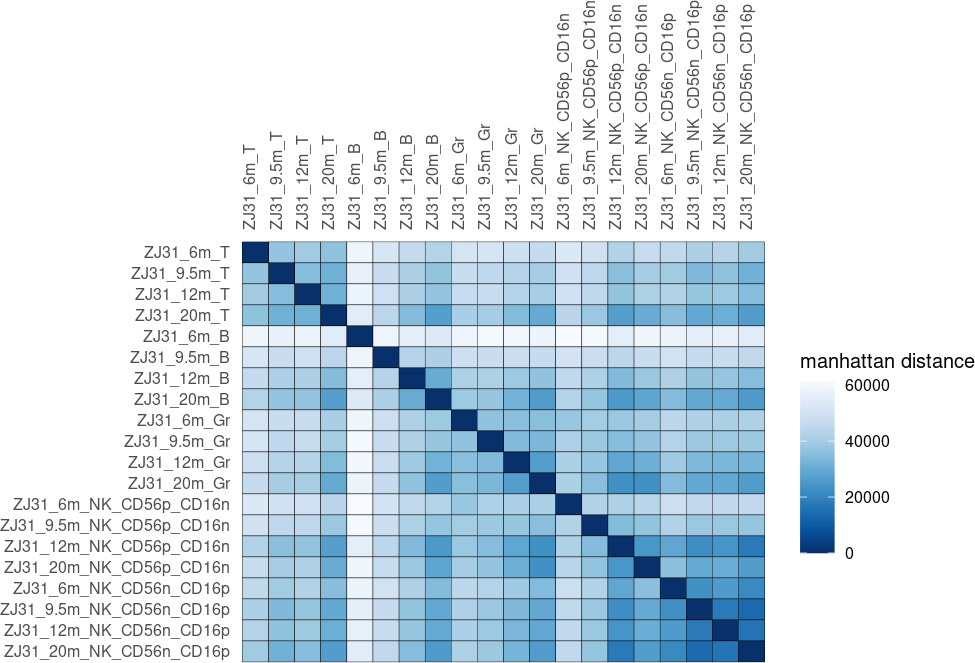Here is the same example, this time using the cosine similarity as the pairwise measure, and imposing a clustering on the resulting similarities (note, in the case of calculating hierarchical clustering on a similarity matrix, we use `proxy` to convert similarities to distances prior to clustering). We can also pick a number of color scales, here choosing “Greens”.

``````dist_plot(wu_SE[,wu_cor_plot_sample_selection],
dist_method =  "cosine",
plot_type = "color",
assay = "logs",
color_pal = "Greens",
cluster_tree = TRUE)``````